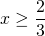# Solution assignment 04 Quadratic inequalities

### Assignment 4

Solve:### Solution

First we solve the corresponding equation: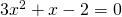and use the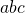-formula: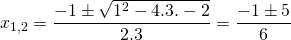Thus the solutions are: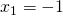or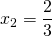The graph of the function in the left-hand side is an 'opens up' parabola and thus the following values ofsatisfy the inequality: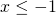or